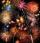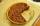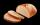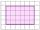Fractions - examples - page 13

1. Product of two fractionsProduct of two fractions is 9 3/5 . If one of the fraction is 9 3/7. Find the other fraction.
2. Equilateral triangle ABCIn the equilateral triangle ABC, K is the center of the AB side, the L point lies on one-third of the BC side near the point C, and the point M lies in the one-third of the side of the AC side closer to the point A. Find what part of the ABC triangle conta
3. UN 1If we add to an unknown number his quarter, we get 210. Identify unknown number.
4. King's birthdayTo celebrate the king's birthday workers fire 1/5 all purchased rockets. To celebrate the Queen's birthday fire 1/6 of the remaining rockets and to celebrate the birthday of king's son remaining 15,000 rockets. How many rockets they purchased?
5. Boys and girlsThere are 28 girls in the hall. 5/7 of all children are boys. How many children and how many boys are there?
6. StudyFor 7 hours study, she scored a 75 so if she made 93 how many hours would she need to study?
7. Series and sequencesFind a fraction equivalent to the recurring decimal? 0.435643564356
8. Right triangle from axesA line segment has its ends on the coordinate axes and forms with them a triangle of area equal to 36 sq. Units . The segment passes through the point ( 5,2). What is the slope of the line segment. ?
9. Points on line segmentPoints P & Q belong to segment AB. If AB=a, AP = 2PQ = 2QB, find the distance: between point A and the midpoint of the segment QB.
10. PieVili ate three pieces of pie . If it piece is 1/8 how much pie did he eat?
11. Unknown numberI think the number - its sixth is 3 smaller than its third.
12. Pie IIVili ate three pieces of pie. If it pieces is 1/8 how much pie did he eat?
13. PearsThere were pears in the basket, I took two-fifths of them, and left six in the basket. How many pears did I take?
14. Percentages 5\$5.25 is 7 1/2% of what number?Several pieces of bread were in the store. Half of them plus a half of bread bought a cookbook. Of the remaining bread, half of it plus half of the bread was bought by Mr. Novák. The last bread was bought by Ms. Small. How many breads were in the store at.
16. Unit rateFind unit rate: 6,840 customers in 45 days
17. PercentagesWhat is 1/3 of \$821.43?
18. Equation with xSolve the following equation: 2x- (8x + 1) - (x + 2) / 5 = 9
19. Two brothersThe two brothers were to be divided according to the will of land at an area of 1ha 86a 30m2 in a ratio of 5:4. How many will everyone get?Shade the area on the grid that shows 5/8 x 2/4